# How To: Calculate Average Linear Velocity Or Speed

In 2A1 Mechanics we really just deal with the vector quantity velocity instead of the scalar quantity speed. However, it’s important to realize that velocity and speed are different. Speed is simply not the velocity without direction.

If you are unsure what vector and scalar quantities are, check out this Khan Academy video: Introduction to Vectors and Scalars

Remember that velocity being a vector quantity has magnitude and displacement. Speed being a scalar quantity only has magnitude and no direction.

This means when describing the vector quantity velocity of your vehicle you would say 100km/hr “magnitude” to the north “direction”. The direction can be any direction up, down, north, east, west, south, a positive or negative sign in front. What’s important being a vector quantity is that it has magnitude and direction.

When describing the scalar quantity speed of your vehicle you would say 100km/hr. Only the magnitude of the vehicle is given, no direction of movement is provided.

## Definitions

Average Velocity: The change in position “displacement” over the change in time. Remember that displacement has magnitude and direction.

Average Speed: Distance travelled over time. Remember that distance has magnitude only and no direction.

## Average Velocity Or Speed Formulas

#### Velocity

The formula to determine average velocity: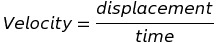Or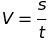Another way to think about it: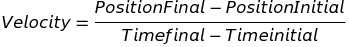The formula for average velocity when an object increases from an initial velocity to a final velocity can be determined with the formula below: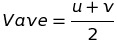#### Speed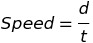Note: Speed uses distance, not displacement. Displacement has both magnitude and direction. Distance has only magnitude and no direction.

## Formula Variables

s = displacement (m)

t = time (s)

u = initial velocity (m/s)

v = final velocity (m/s)

d = distance (m)

## Summary

Here are a couple links which helped me understand how to complete average linear velocity or speed questions.

Recommendation #1

I found this video very helpful. He’s a great teacher and was interesting enough to help me pay attention throughout a long session of studying.

Video: Flipping Physics: Introduction to Velocity and Speed and the differences between the two.

Recommendation #2

Great explanation and provides a couple practice questions with answers.

Website: Physics Classroom: Speed and Velocity